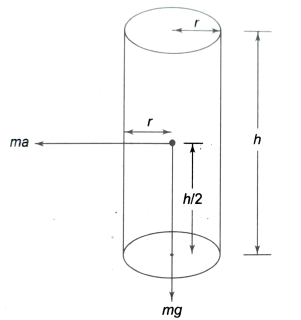Rotational motion
Question

# The height of a solid cylinder is four times its radius, lt is kept vertically at time t = 0 on a belt which is moving in the horizontal direction with a velocity v = 2.45 t2 where v is in ms-1 and t is in second. If the cylinder does not slip, it will topple over at time t (in seconds) equal to…….[ Take  ]

Moderate
Solution

## The cylinder will topple when the torque mgr equals the torque $\mathrm{ma}\frac{\mathrm{h}}{2}$ (see figure)or   …….(i)Now $\mathrm{v}=2.45{\mathrm{t}}^{2}$  ……..(ii)Equating (i) and (ii), we get $\mathrm{t}=\frac{\mathrm{g}}{2×4.9}=\frac{9.8}{9.8}=1\mathrm{s}$

Get Instant Solutions